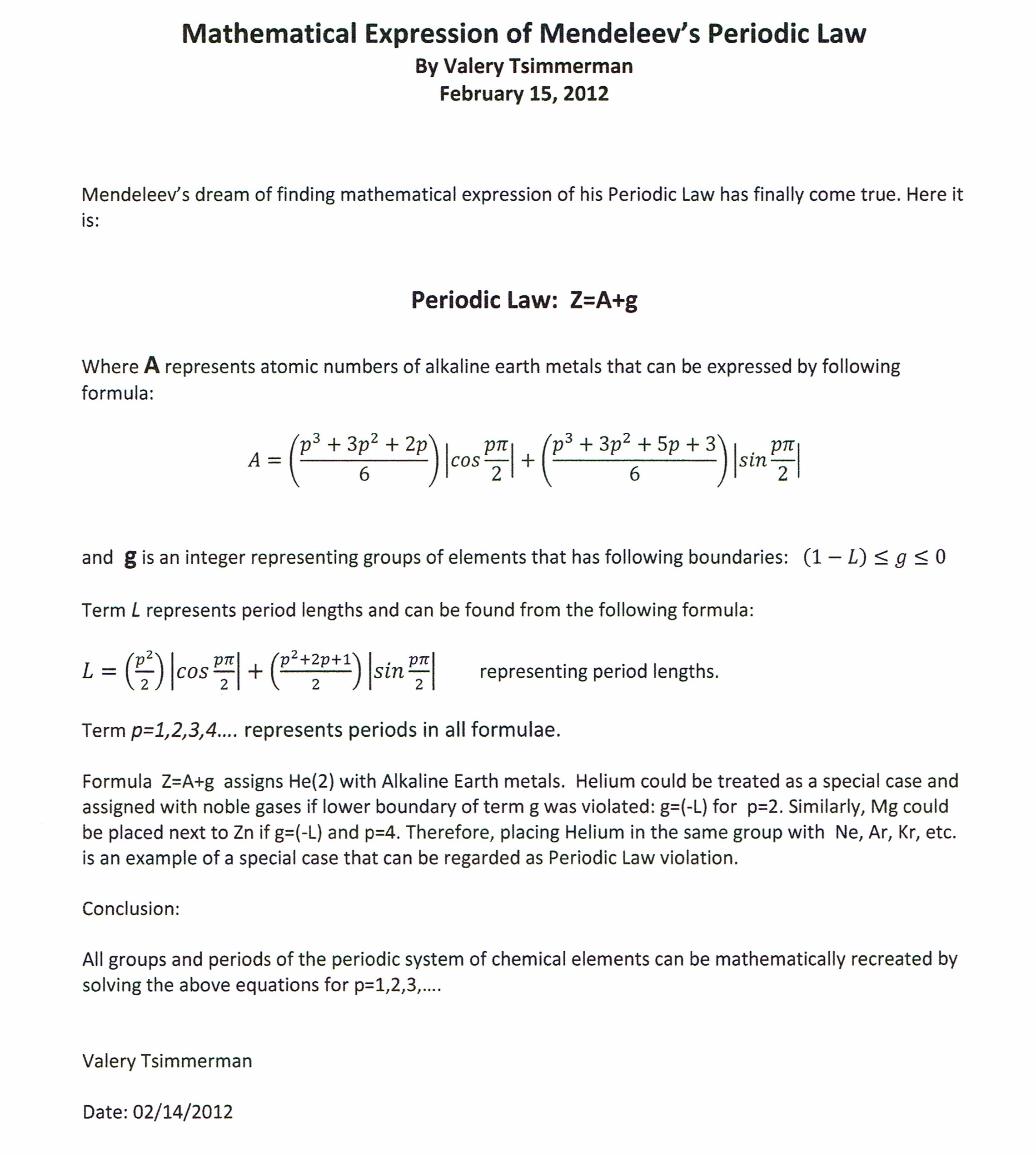### Realization of Tetrahedral Nature of The Periodic System Provided Unique Opportunity for Derivation of the Periodic Law Equation.The algebraic expression Z=A+g (where "Z" is atomic number) is based on an observation that group of alkaline earth metals (described by term "A") plays the role of the back bone in the periodic system. All other groups of elements can be derived by the addition of negative integers (represented by term "g") to the atomic numbers of the alkaline earth metals. The atomic numbers of the alkaline earth metals can be obtained by solving the periodic function "A" for p= 1,2,3,4..., period numbers.

The alkaline earth function "A" consists of two parts, first of which is the tetrahedral number formula that describes atomic numbers of all alkaline earth metals located in even periods and the second is the tetrahedral number formula plus (p+1)/2, that describes all alkaline earths located in odd periods.

Expression Z=A+g accurately captures position of all known chemical elements in their respective groups found in the Periodic Table with the exception of Helium (Z=2), which in traditional periodic table is located in the same group with the elements known as noble gases. Despite being called a noble gas, Helium has the spectroscopic signature of an alkaline earth metal. The Mathematical Expression of the Periodic Law presented here provides an unequivocal argument that the current classification of Helium as a noble gas is at odds with the rest of the Periodic System.

Below is another version of the Periodic Law Equation that does not use trigonometric functions for description of period lengths and atomic numbers of alkaline earth metals:It can be stated that the elements belong to the same period if they correspond to the same value of "p". The elements belong to the same group if they correspond to the same value of "g".

Home